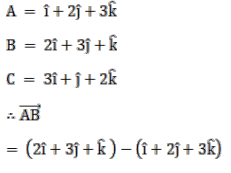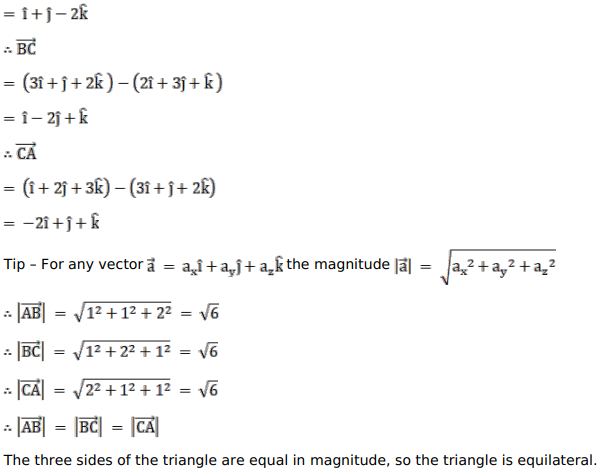# If the position vectors of the vertices`
Question:

If the position vectors of the vertices $A, B$ and $C$ of a $\triangle A B C$ be $(\hat{i}+2 \hat{j}+3 \hat{k}),(2 \hat{i}+3 \hat{j}+\hat{k})$ and $(3 \hat{i}+\hat{j}+2 \hat{k})$ respectively, prove that $\triangle A B C$ is equilateral.

Solution: